# Linked List Basic Concepts A list refers to

• Slides: 55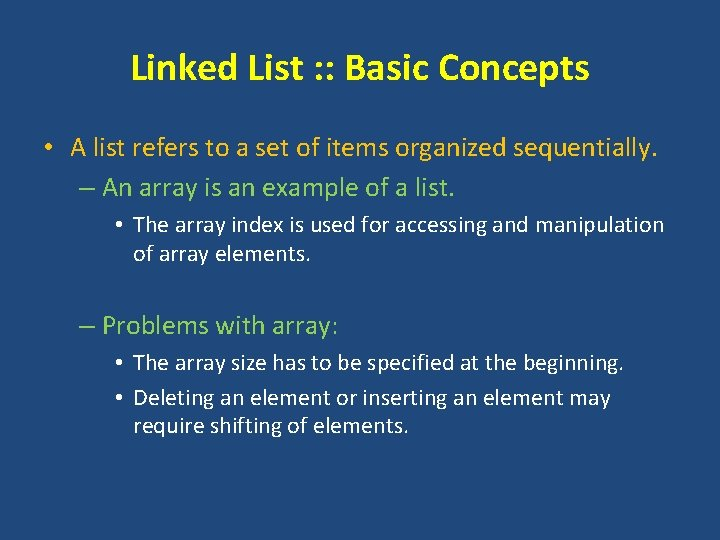Linked List : : Basic Concepts • A list refers to a set of items organized sequentially. – An array is an example of a list. • The array index is used for accessing and manipulation of array elements. – Problems with array: • The array size has to be specified at the beginning. • Deleting an element or inserting an element may require shifting of elements.Linked List Structure Variable 1 item Structure Variable 2 item Structure Variable 3 item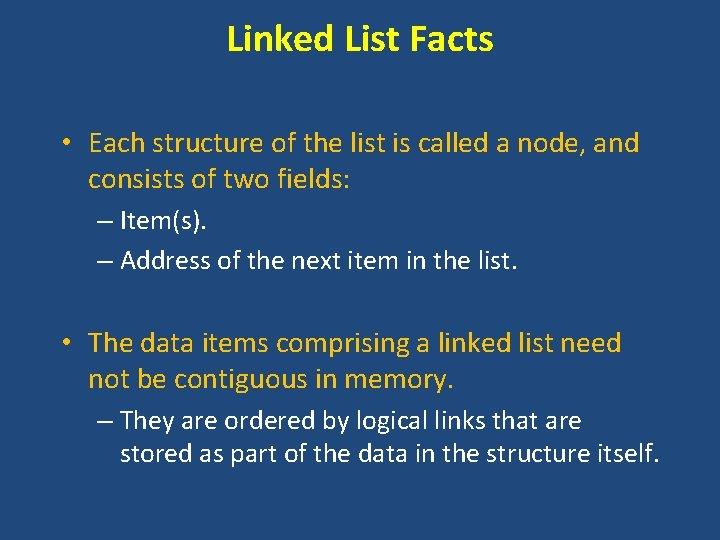Linked List Facts • Each structure of the list is called a node, and consists of two fields: – Item(s). – Address of the next item in the list. • The data items comprising a linked list need not be contiguous in memory. – They are ordered by logical links that are stored as part of the data in the structure itself.Declaration of a linked list struct node { int item; struct node *next; }; node item next • Such structures which contain a member field pointing to the same structure type are called self-referential structures.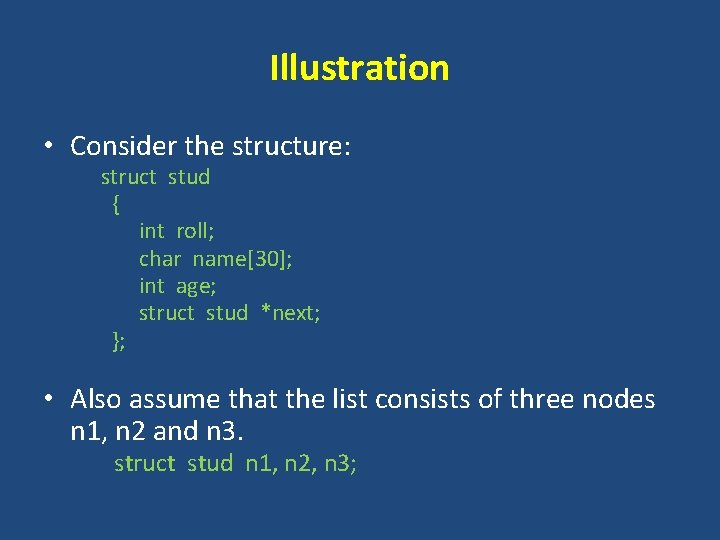Illustration • Consider the structure: struct stud { int roll; char name; int age; struct stud *next; }; • Also assume that the list consists of three nodes n 1, n 2 and n 3. struct stud n 1, n 2, n 3;Illustration • To create the links between nodes, we can write: n 1. next = &n 2 ; n 2. next = &n 3 ; n 3. next = NULL ; /* No more nodes follow */ • Now the list looks like: roll name age next n 1 n 2 n 3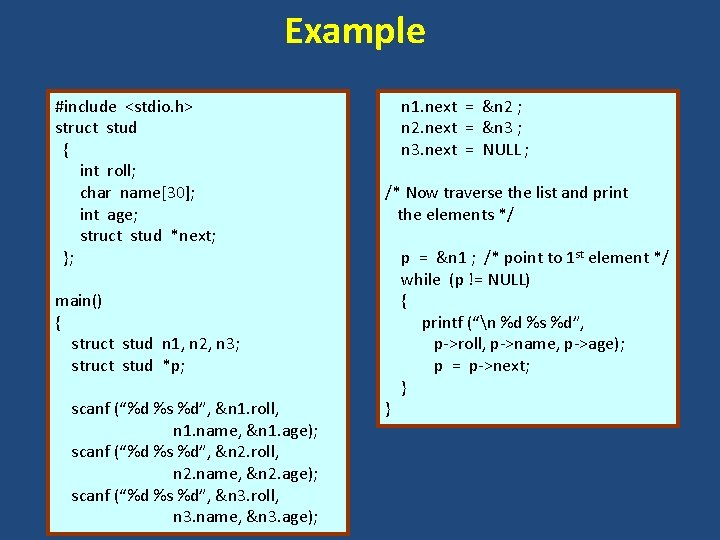Example #include <stdio. h> struct stud { int roll; char name; int age; struct stud *next; }; n 1. next = &n 2 ; n 2. next = &n 3 ; n 3. next = NULL ; /* Now traverse the list and print the elements */ main() { struct stud n 1, n 2, n 3; struct stud *p; scanf (“%d %s %d”, &n 1. roll, n 1. name, &n 1. age); scanf (“%d %s %d”, &n 2. roll, n 2. name, &n 2. age); scanf (“%d %s %d”, &n 3. roll, n 3. name, &n 3. age); } p = &n 1 ; /* point to 1 st element */ while (p != NULL) { printf (“n %d %s %d”, p->roll, p->name, p->age); p = p->next; }Linked List • Where to start and where to stop? Head / Start Structure Variable 1 item Structure Variable 2 item Structure Variable 3 item End / Last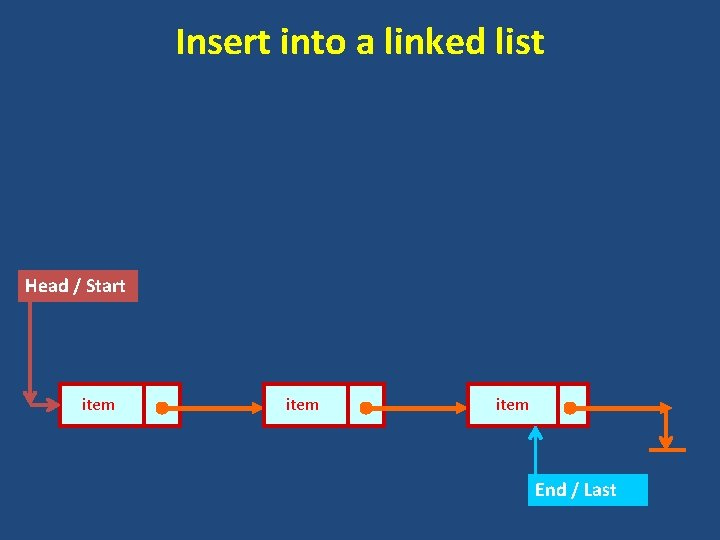Insert into a linked list Head / Start item End / Last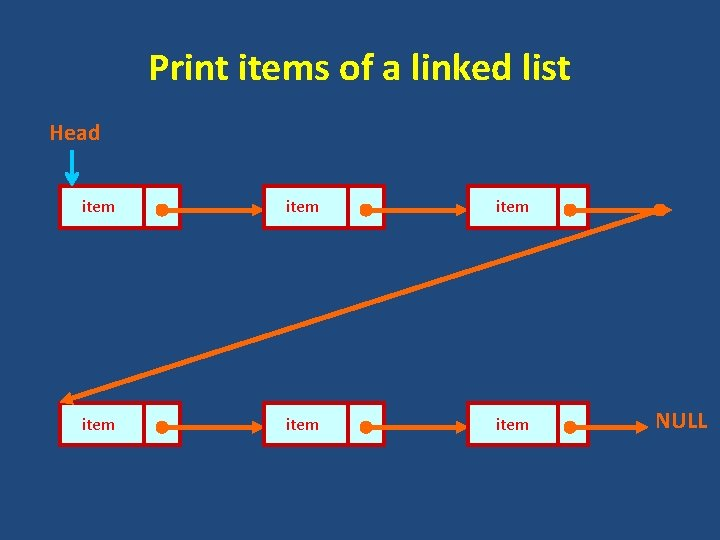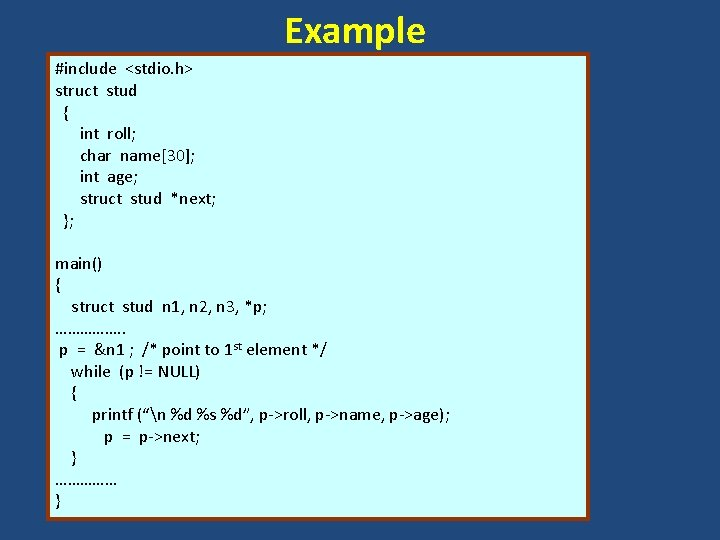Example #include <stdio. h> struct stud { int roll; char name; int age; struct stud *next; }; main() { struct stud n 1, n 2, n 3, *p; ……………. . p = &n 1 ; /* point to 1 st element */ while (p != NULL) { printf (“n %d %s %d”, p->roll, p->name, p->age); p = p->next; } …………… }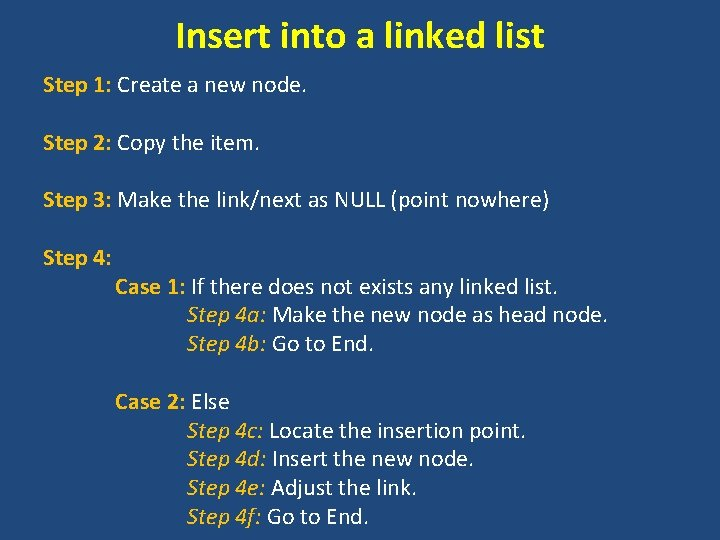Insert into a linked list Step 1: Create a new node. Step 2: Copy the item. Step 3: Make the link/next as NULL (point nowhere) Step 4: Case 1: If there does not exists any linked list. Step 4 a: Make the new node as head node. Step 4 b: Go to End. Case 2: Else Step 4 c: Locate the insertion point. Step 4 d: Insert the new node. Step 4 e: Adjust the link. Step 4 f: Go to End.Insert into a linked list item NULL Step 1: Create a new node. Step 2: Copy the item. Step 3: Make the link/next as NULL (point nowhere) Step 4: Case 1: If there does not exists any linked list. Step 4 a: Make the new node as head node. Step 4 b: Go to End.Insert into a linked list item NULL Head item Step 1: Create a new node. Step 2: Copy the item. Step 3: Make the link/next as NULL (point nowhere) Step 4: Case 2: Else Step 4 c: Locate the insertion point. Step 4 d: Insert the new node. Step 4 e: Adjust the link. Step 4 f: Go to End. item NULLInsert into a linked list: End Node item NULL Head item Step 4 ci: Traverse till last/end node. Step 4 cii: Make the next of last node as the address of new node. NULL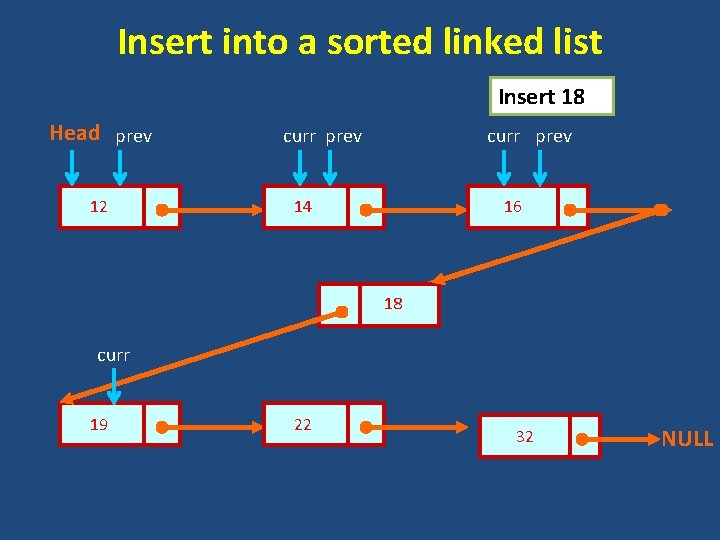Insert into a sorted linked list Insert 18 Head prev 12 curr prev 14 16 18 curr 19 22 32 NULLDelete a specific node from linked list Delete 18 Head 12 prev 14 16 18 curr 19 22 32 NULL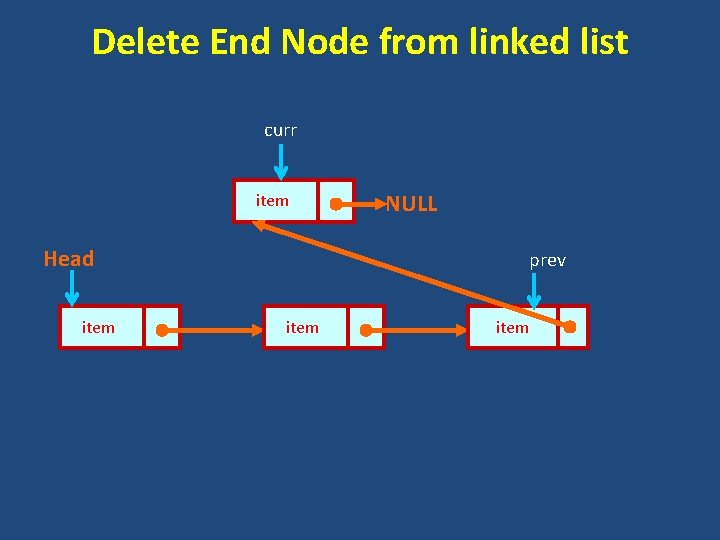Delete End Node from linked list curr item Head item NULL prev item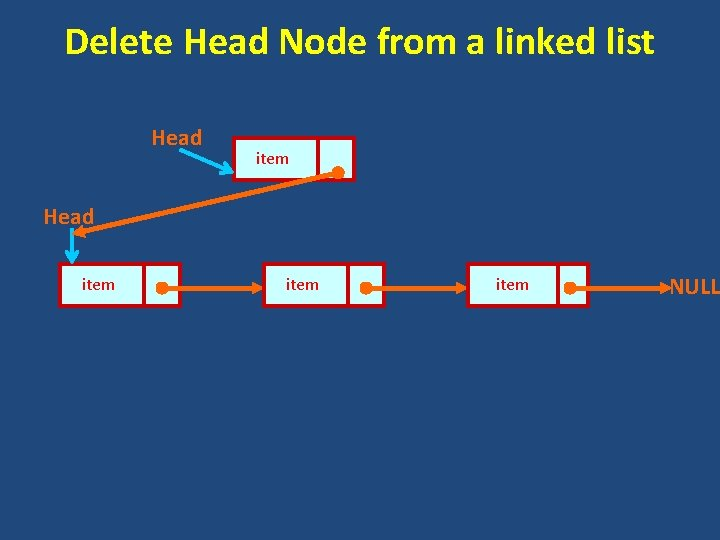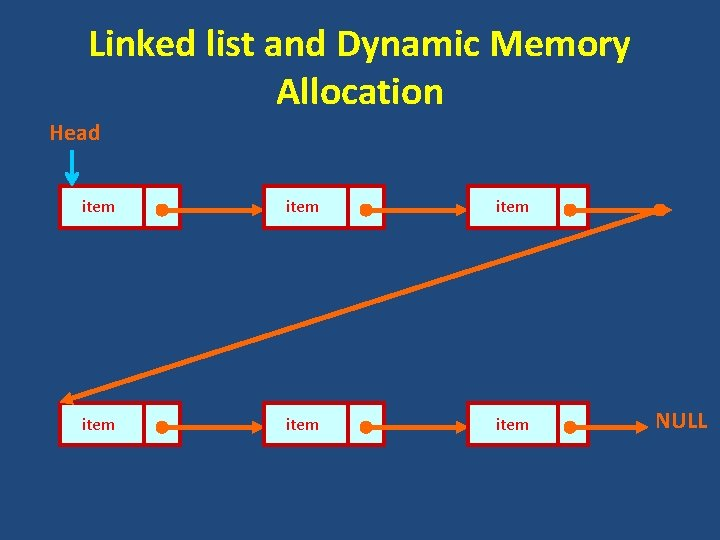Linked list and Dynamic Memory Allocation 1. We need not know how many nodes are there. 2. Dynamic memory allocation provides a flexibility on the length of a linked list. 3. Example, struct node { int data; struct node *next; }; struct node *head, *temp; temp=(struct node *)malloc(sizeof(struct node)); temp->next=NULL; temp->data=10; head=temp; free(temp);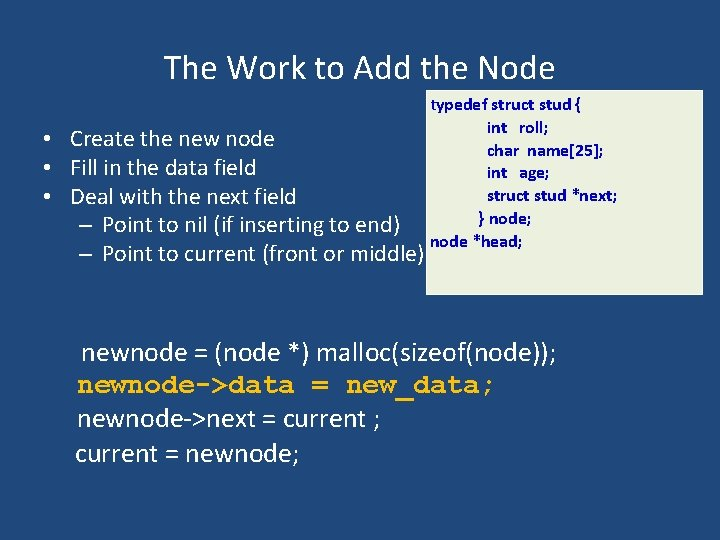The Work to Add the Node • Create the new node • Fill in the data field • Deal with the next field – Point to nil (if inserting to end) – Point to current (front or middle) typedef struct stud { int roll; char name; int age; struct stud *next; } node; node *head; newnode = (node *) malloc(sizeof(node)); newnode->data = new_data; newnode->next = current ; current = newnode;Three Types of Insertion • front • end – Get to the end, then add node • In middle – Get to the node before which the new node is to be inserted.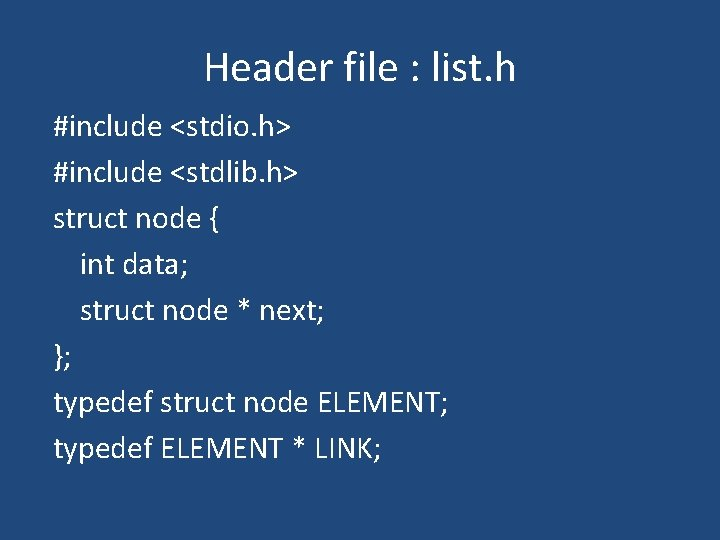Header file : list. h #include <stdio. h> #include <stdlib. h> struct node { int data; struct node * next; }; typedef struct node ELEMENT; typedef ELEMENT * LINK;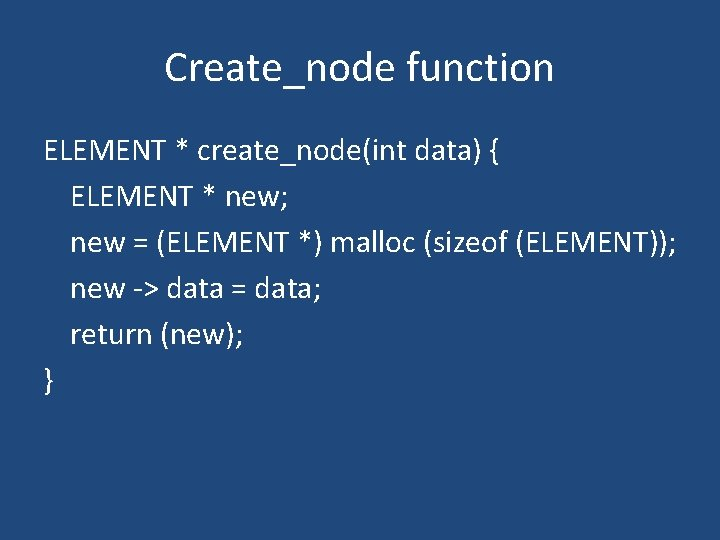Create_node function ELEMENT * create_node(int data) { ELEMENT * new; new = (ELEMENT *) malloc (sizeof (ELEMENT)); new -> data = data; return (new); }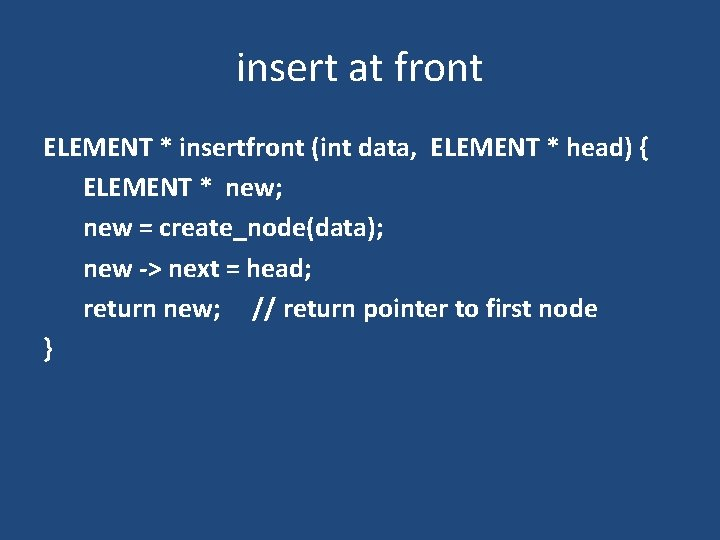insert at front ELEMENT * insertfront (int data, ELEMENT * head) { ELEMENT * new; new = create_node(data); new -> next = head; return new; // return pointer to first node }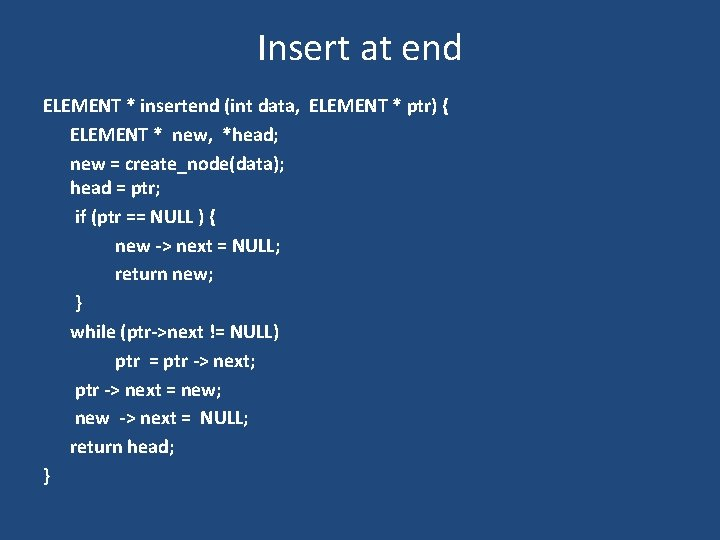Insert at end ELEMENT * insertend (int data, ELEMENT * ptr) { ELEMENT * new, *head; new = create_node(data); head = ptr; if (ptr == NULL ) { new -> next = NULL; return new; } while (ptr->next != NULL) ptr = ptr -> next; ptr -> next = new; new -> next = NULL; return head; }Sorted insert function ELEMENT * insert (int data, ELEMENT * ptr) { LINK new, prev, first; new = create_node(data); if (ptr == NULL || data < ptr -> value){ // insert as new first node new -> next = ptr; return new; // return pointer to first node }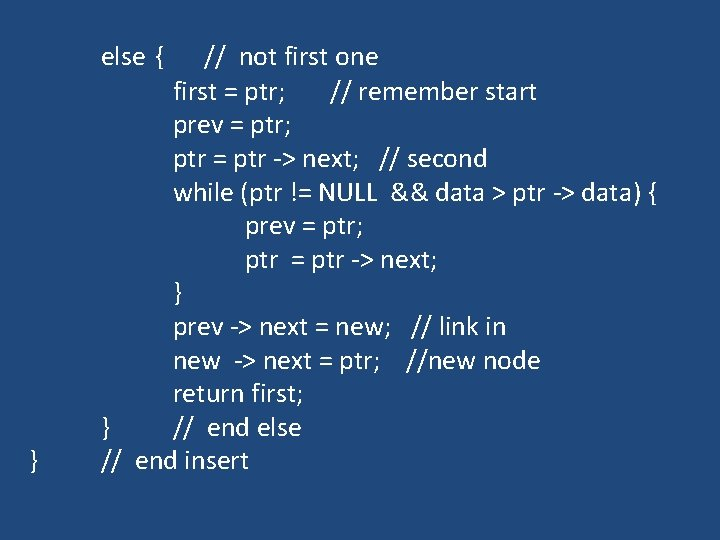else { } // not first one first = ptr; // remember start prev = ptr; ptr = ptr -> next; // second while (ptr != NULL && data > ptr -> data) { prev = ptr; ptr = ptr -> next; } prev -> next = new; // link in new -> next = ptr; //new node return first; } // end else // end insertDeletion To delete a data item from a linked list involves (assuming it occurs only once!): • finding the data item in the list, and • linking out this node, and • freeing up this node as free space.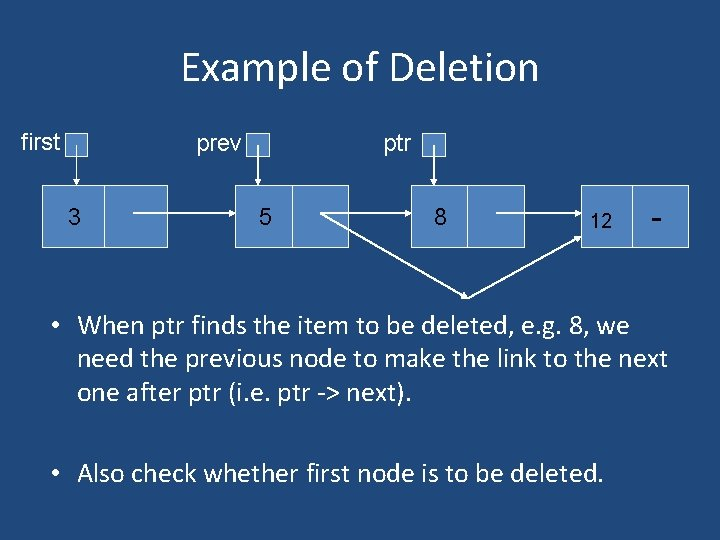Example of Deletion first prev 3 ptr 5 8 12 - • When ptr finds the item to be deleted, e. g. 8, we need the previous node to make the link to the next one after ptr (i. e. ptr -> next). • Also check whether first node is to be deleted.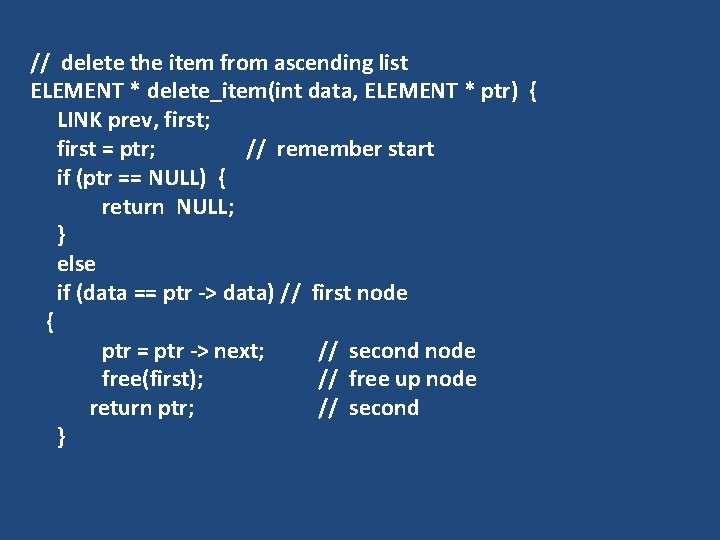// delete the item from ascending list ELEMENT * delete_item(int data, ELEMENT * ptr) { LINK prev, first; first = ptr; // remember start if (ptr == NULL) { return NULL; } else if (data == ptr -> data) // first node { ptr = ptr -> next; // second node free(first); // free up node return ptr; // second }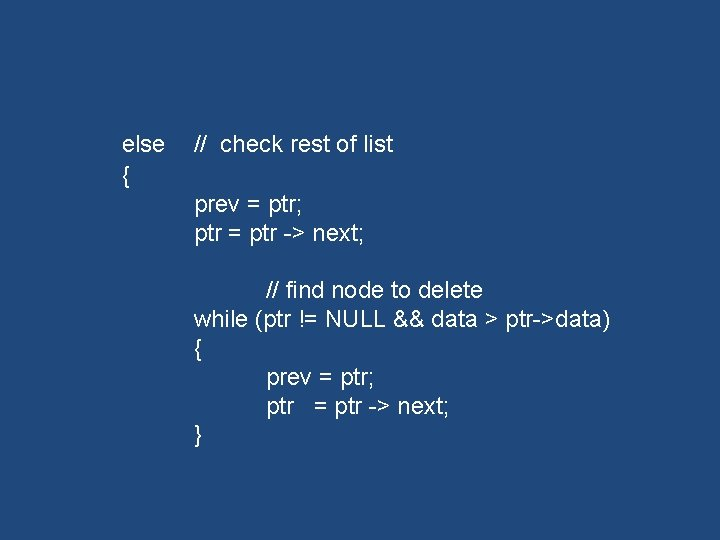else { // check rest of list prev = ptr; ptr = ptr -> next; // find node to delete while (ptr != NULL && data > ptr->data) { prev = ptr; ptr = ptr -> next; }if (ptr == NULL || data != ptr->data) // NOT found in ascending list // nothing to delete { return first; // original } else // found, delete ptr node { prev -> next = ptr -> next; free(ptr); // free node return first; // original } } } // end delete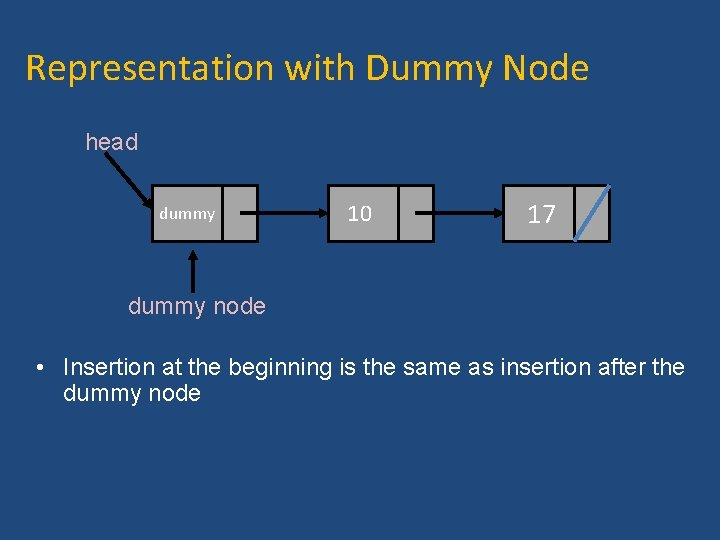Representation with Dummy Node head dummy 10 17 dummy node • Insertion at the beginning is the same as insertion after the dummy nodeInitialization head Write a function that initializes LIST typedef struct list { int data; struct list *next; } ELEMENT; ELEMENT* Initialize (int element) { ELEMENT *head; head = (ELEMENT *)malloc(sizeof(data)); // Create initial node head->data = element; head -> next = NULL; return head; }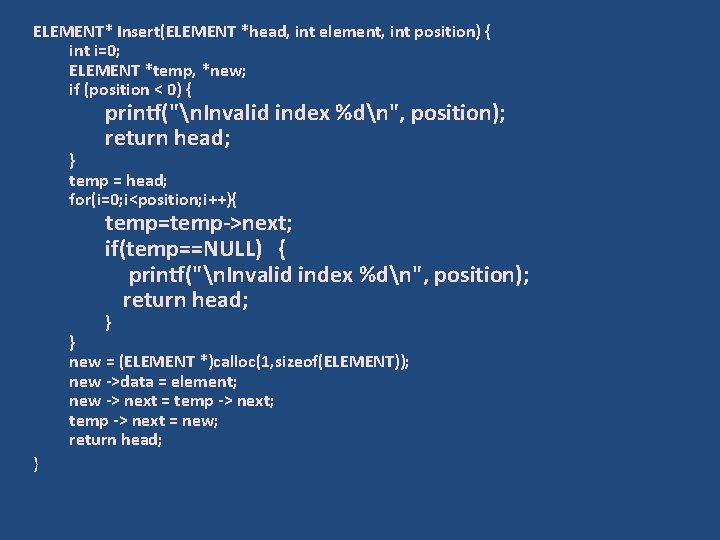ELEMENT* Insert(ELEMENT *head, int element, int position) { int i=0; ELEMENT *temp, *new; if (position < 0) { printf("n. Invalid index %dn", position); return head; } temp = head; for(i=0; i<position; i++){ temp=temp->next; if(temp==NULL) { printf("n. Invalid index %dn", position); return head; } } new = (ELEMENT *)calloc(1, sizeof(ELEMENT)); new ->data = element; new -> next = temp -> next; temp -> next = new; return head; }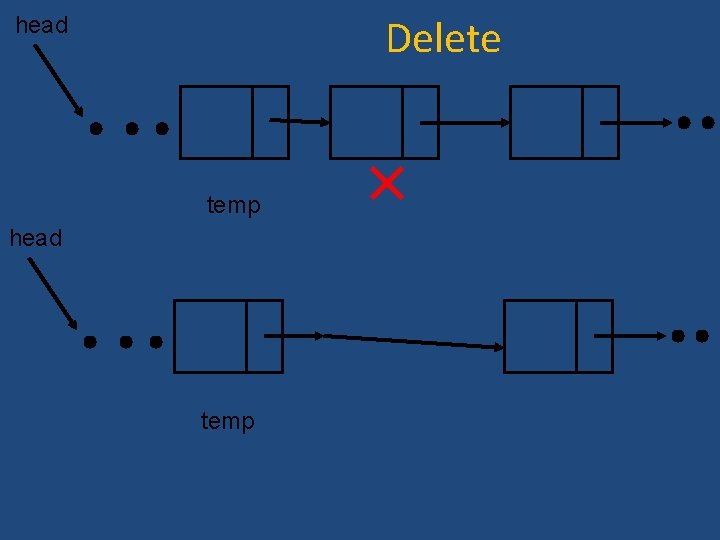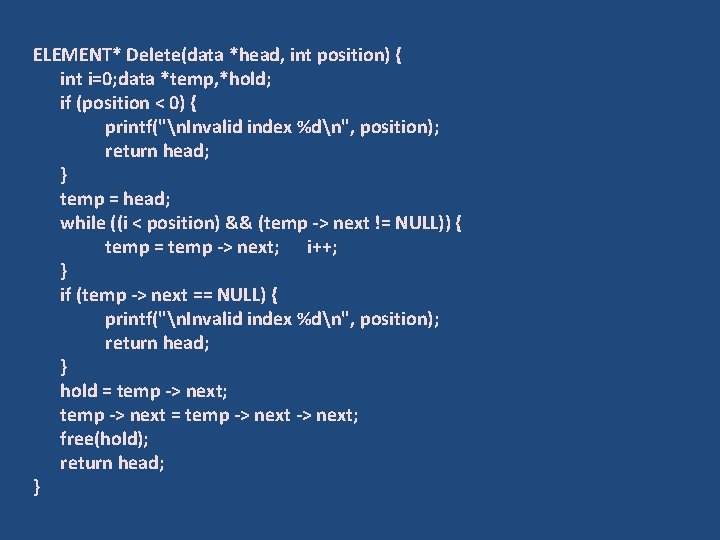ELEMENT* Delete(data *head, int position) { int i=0; data *temp, *hold; if (position < 0) { printf("n. Invalid index %dn", position); return head; } temp = head; while ((i < position) && (temp -> next != NULL)) { temp = temp -> next; i++; } if (temp -> next == NULL) { printf("n. Invalid index %dn", position); return head; } hold = temp -> next; temp -> next = temp -> next; free(hold); return head; }Linked list and Dynamic Memory Allocation 1. We need not know how many nodes are there. 2. Dynamic memory allocation provides a flexibility on the length of a linked list. 3. Example, struct node { int item; struct node *next; }; struct node *head, *temp; temp=(struct node *)malloc(sizeof(struct node)*1); temp->next=NULL; temp->item=10; head=temp; free(temp);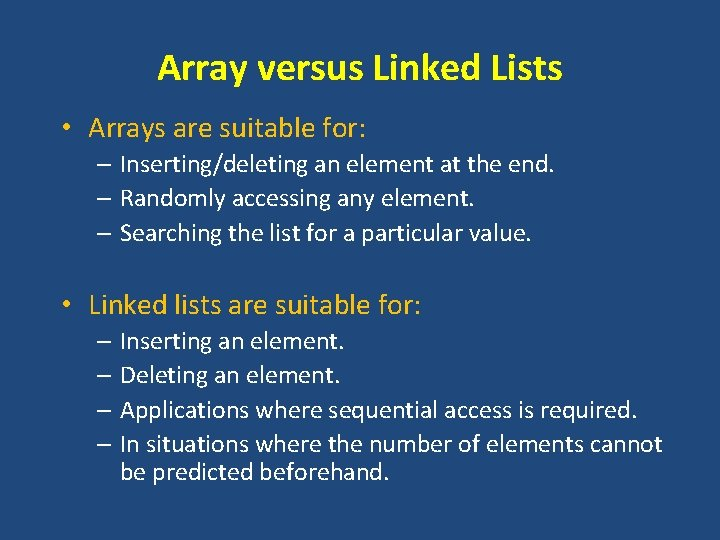Array versus Linked Lists • Arrays are suitable for: – Inserting/deleting an element at the end. – Randomly accessing any element. – Searching the list for a particular value. • Linked lists are suitable for: – Inserting an element. – Deleting an element. – Applications where sequential access is required. – In situations where the number of elements cannot be predicted beforehand.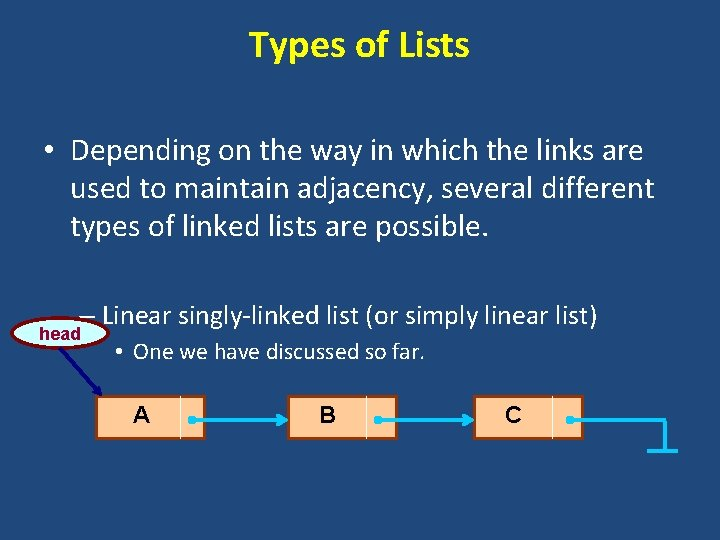Types of Lists • Depending on the way in which the links are used to maintain adjacency, several different types of linked lists are possible. – Linear singly-linked list (or simply linear list) head • One we have discussed so far. A B C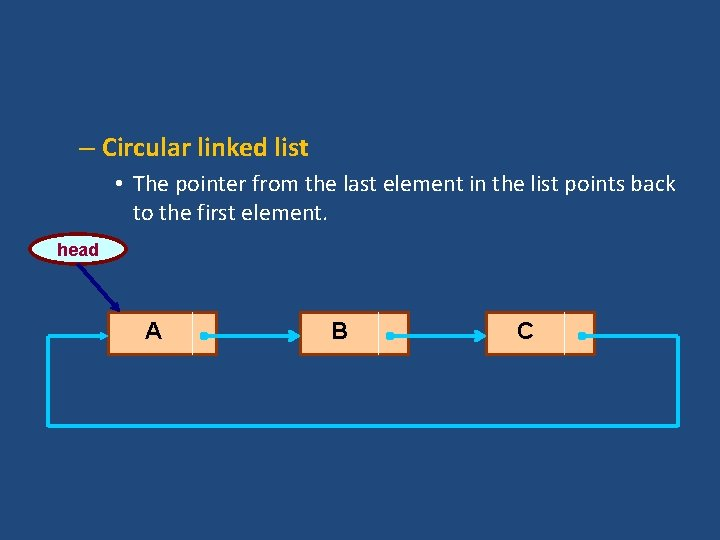– Circular linked list • The pointer from the last element in the list points back to the first element. head A B C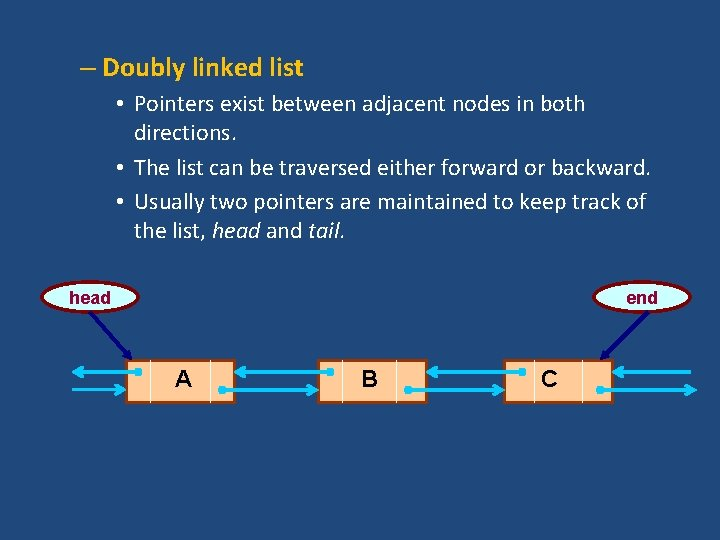– Doubly linked list • Pointers exist between adjacent nodes in both directions. • The list can be traversed either forward or backward. • Usually two pointers are maintained to keep track of the list, head and tail. head end A B C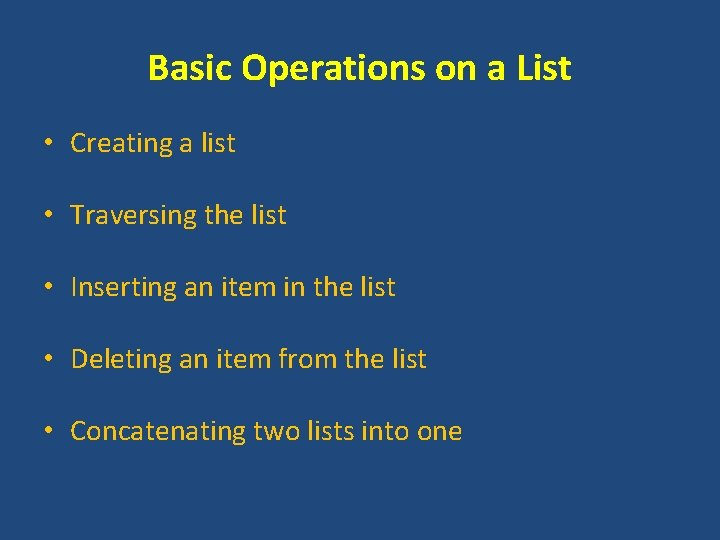Basic Operations on a List • Creating a list • Traversing the list • Inserting an item in the list • Deleting an item from the list • Concatenating two lists into one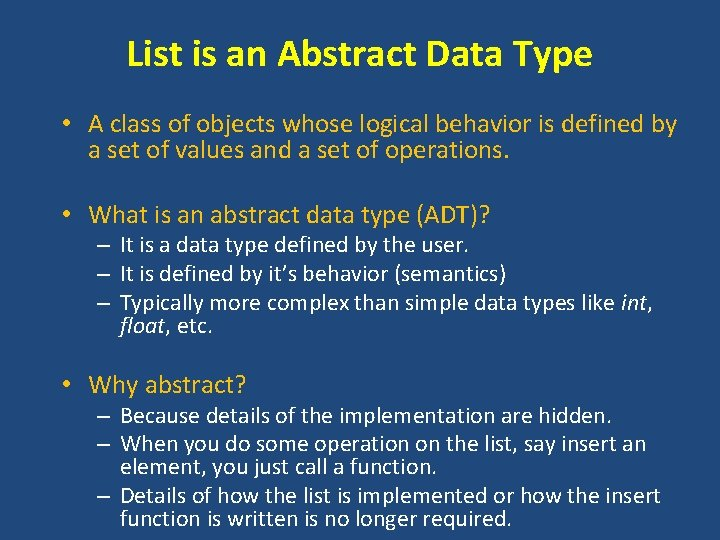List is an Abstract Data Type • A class of objects whose logical behavior is defined by a set of values and a set of operations. • What is an abstract data type (ADT)? – It is a data type defined by the user. – It is defined by it’s behavior (semantics) – Typically more complex than simple data types like int, float, etc. • Why abstract? – Because details of the implementation are hidden. – When you do some operation on the list, say insert an element, you just call a function. – Details of how the list is implemented or how the insert function is written is no longer required.Example Write a C Program to store student course information using structures with Dynamically Memory Allocation. Input: Enter number of records: 2 Enter name of the subject and marks respectively: Programming 22 Enter name of the subject and marks respectively: Structure 33 Output: Displaying Information: Programming 22 Structure 33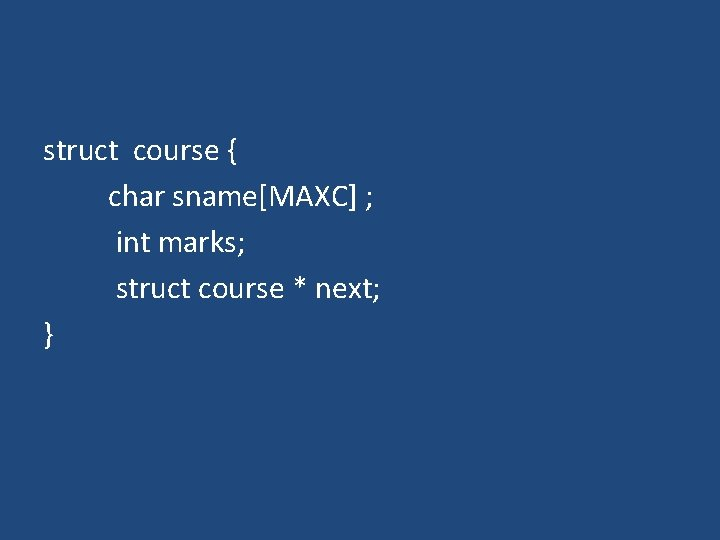struct course { char sname[MAXC] ; int marks; struct course * next; }int main () { int num, i; struct course * head = NULL; struct course * new, *p; printf (“number of courses? ”) ; scanf (“%d”, &num) ; for (i=0; i<num; i++) { new = malloc (sizeof (struct course)) ; new->next = head; scanf (“%s”, new->sname) ; scanf(“%d”, &new->marks); head = new; } p =head; while (p!=NULL) { printf (“%s -- %dn”, p->sname, p->marks) ; p=p->next; } }int main () { int num, i; struct course * head = NULL; struct course * new, *p; printf (“number of courses? ”) ; scanf (“%d”, &num) ; for (i=0; i<num; i++) { new = malloc (sizeof (struct course)) ; if (head==NULL) head = new; else p->next = new; scanf (“%s”, new->sname) ; scanf(“%d”, &new->marks); p = new; } new->next = NULL; p =head; while (p!=NULL) { printf (“%s -- %dn”, p->sname, p->marks) ; p=p->next; } }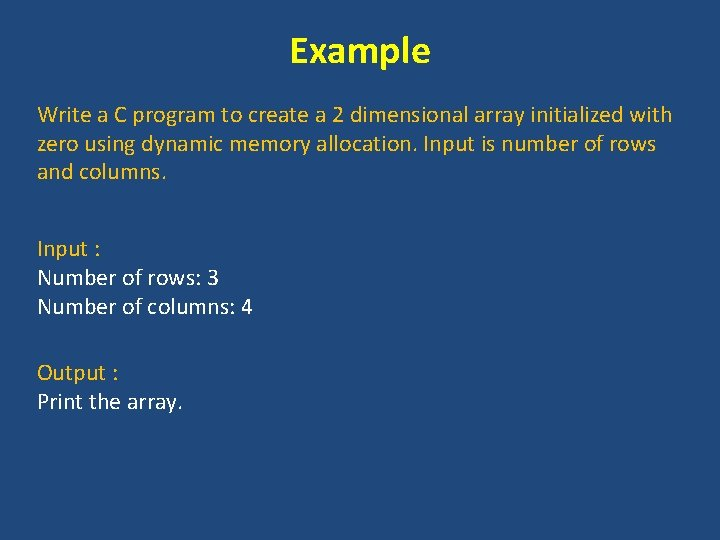Example Write a C program to create a 2 dimensional array initialized with zero using dynamic memory allocation. Input is number of rows and columns. Input : Number of rows: 3 Number of columns: 4 Output : Print the array.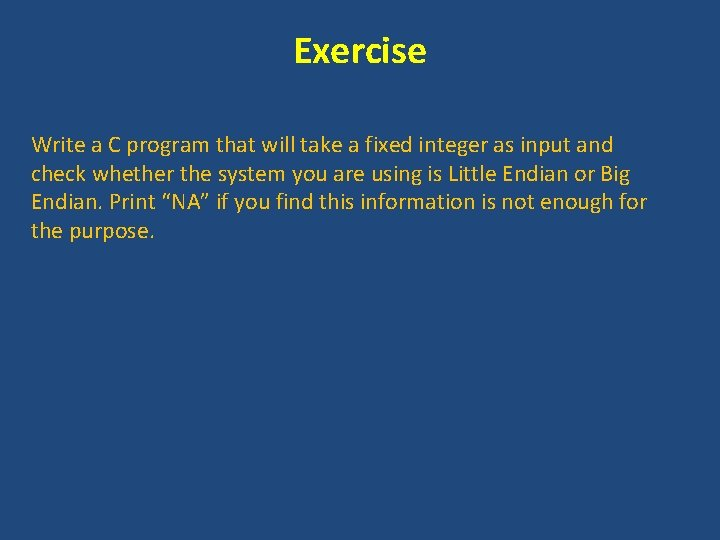Exercise Write a C program that will take a fixed integer as input and check whether the system you are using is Little Endian or Big Endian. Print “NA” if you find this information is not enough for the purpose.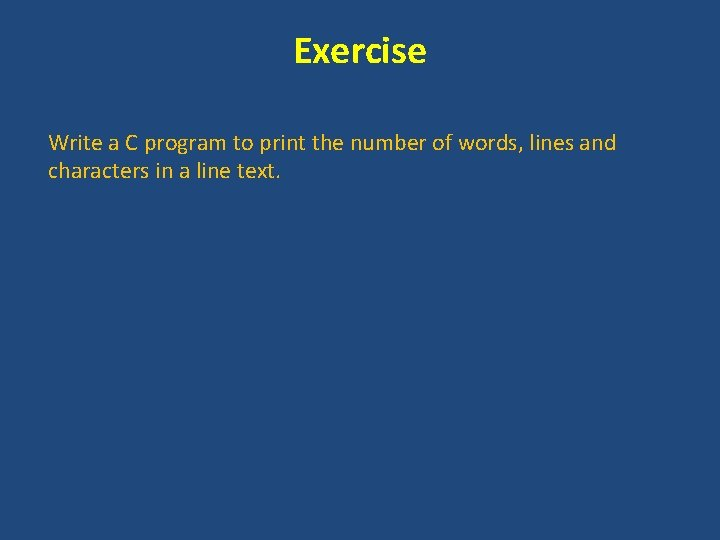Exercise Write a C program to print the number of words, lines and characters in a line text.